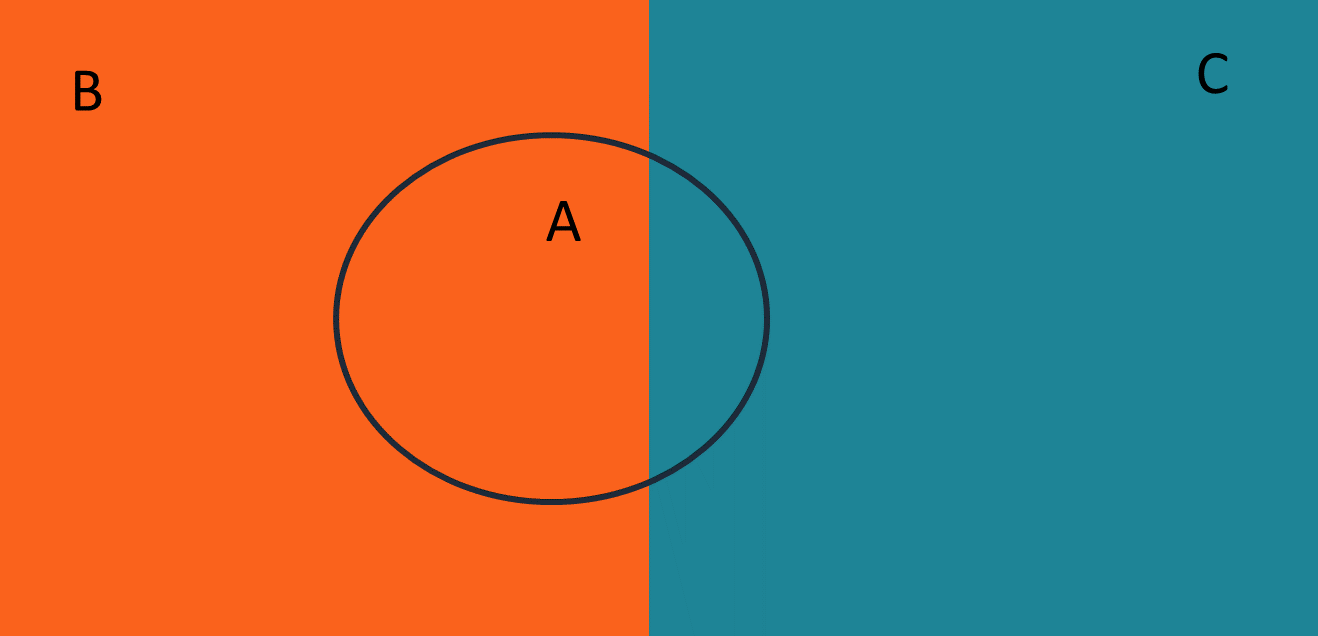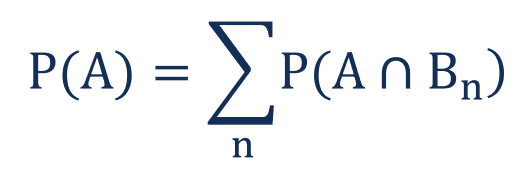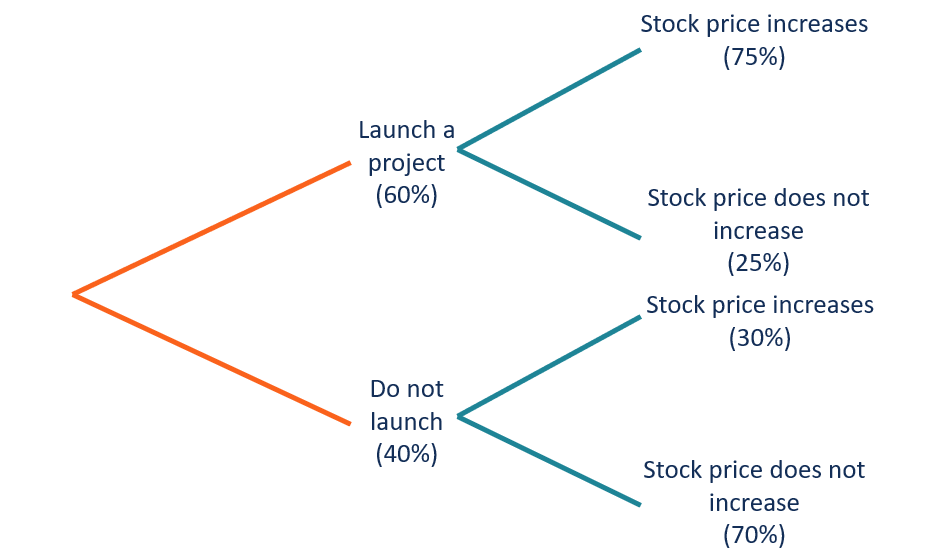# Total Probability Rule

The use of known probabilities of several distinct events to calculate the probability of an event

## What is the Total Probability Rule?

The Total Probability Rule (also known as the Law of Total Probability) is a fundamental rule in statistics relating to conditional and marginal probabilities. The rule states that if the probability of an event is unknown, it can be calculated using the known probabilities of several distinct events.

Consider the situation in the image below:There are three events: A, B, and C. Events B and C are distinct from each other while event A intersects with both events. We do not know the probability of event A. However, we know the probability of event A under condition B and the probability of event A under condition C.

The total probability rule states that by using the two conditional probabilities, we can find the probability of event A (i.e., total probability).

### Formula for the Total Probability Rule

Mathematically, the total probability rule can be written in the following equation:Where:

• n – the number of events
• Bn – the distinct event

Remember that the multiplication probability rule states the following:

##### P(A ∩ B) = P(A|B) × P(B)

For example, the total probability of event A from the situation above can be found using the equation below:

### The Total Probability Rule and Decision Trees

The decision tree is a simple and convenient method of visualizing problems with the total probability rule. The decision tree depicts all possible events in the sequence. Using the decision tree, you can quickly identify the relationships between the events and calculate the conditional probabilities.

In order to understand how to utilize a decision tree for the calculation of the total probability, let’s consider the following example:

You are a stock analyst following ABC Corp. You discovered that the company is planning to launch a new project that is likely to affect the company’s stock price. You identified the following probabilities:

• There is a 60% probability of launching a new project.
• If a company launches the project, there is a 75% probability that its stock price will increase.
• If a company does not launch the project, there is a 30% probability that its stock price will increase.

You want to find the probability that the company’s stock price will increase. The decision tree for the problem is:You need only the probability of the events when the stock price increases. Using the decision tree, we can calculate the following conditional probabilities:

P(Launch a project|Stock price increases) = 0.6 × 0.75 = 0.45

P(Do not launch|Stock price increases) = 0.4 × 0.30 = 0.12

According to the total probability rule, the probability of a stock price increase is:

P(Stock price increases) = P(Launch a project|Stock price increases) + P(Do not launch|Stock price increases)

= 0.45 + 0.12 = 0.57

Thus, there is a 57% probability that the company’s share price will increase.